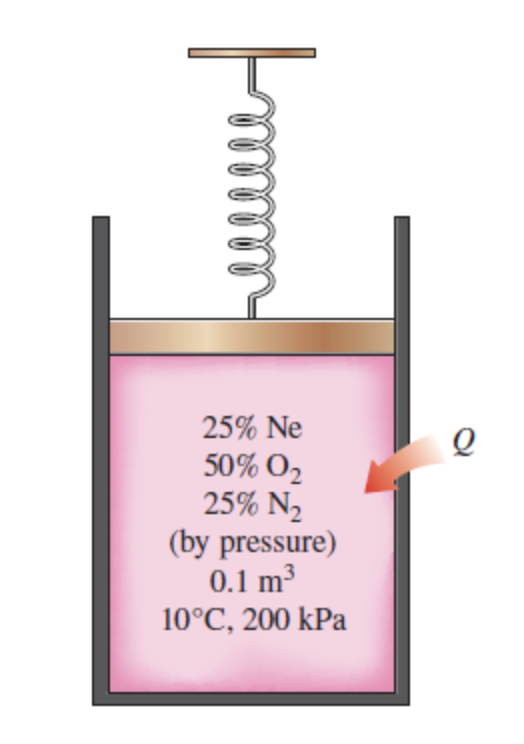25% Ne50% 0225% N,(by pressure)0.1 m310°C, 200 kPa

Question

A spring-loaded piston–cylinder device contains a mixture of gases whose pressure fractions are 25 percent Ne, 50 percent O2, and 25 percent N2. The piston diameter and spring are selected for this device such that the volume is 0.1 m3 when the pressure is 200 kPa and 1.0 m3 when the pressure is 1000 kPa. Initially, the gas is added to this device until the pressure is 200 kPa and the temperature is 10°C. The device is now heated until the pressure is 550 kPa. Calculate the total work and heat transfer for this process. The universal gas constant is Ru = 8.314 kJ/kmol·K. Use the table containing the ideal-gas specific heats of various common gases and the table containing the molar mass, gas constant, and critical-point properties.

The total work for this process is _________kJ.

The heat transfer for this process is __________kJ.help_outlineImage Transcriptionclose25% Ne 50% 02 25% N, (by pressure) 0.1 m3 10°C, 200 kPa fullscreen
Step 1

Given Information:

Pressure fraction of Neon

Pressure fraction of Oxygen

Pressure fraction of Nitrogen

P1= 550 kPa

P2=200 KPa

Step 2

From the table,

The molar masses of

The molar masses of

The molar masses of

And gas constants for Neon, oxygen and nitrogen are:

From the table,

The molar masses of Ne=20.18 KJ/Kmol

The molar masses of O2= 32KJ/Kmol

The molar masses of N2= 28KJ/Kmol

And gas constants for Neon, oxygen and nitrogen are:

The constant-volume specific heats for Neon, oxygen and nitrogen are:

Step 3

At the given pressure  the partial pressure of all the components are:

The mass of...

Want to see the full answer?

See Solution

Want to see this answer and more?

Our solutions are written by experts, many with advanced degrees, and available 24/7

See Solution
Tagged in

Mechanical Engineering